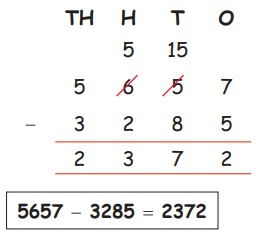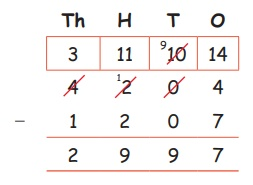Home | | Maths 4th Std | Subtraction with Regrouping

# Subtraction with Regrouping

Subtraction with Regrouping.

Subtraction with Regrouping.

EXAMPLES

1. Subtract 3285 from 5657

Step: 1

Arrange the numbers in columns as shown below.Step 1: It is not possible to subtract 8 tens from 5 tens. So, borrow 1 hundred from the hundreds column.

Step 2: 6 hundreds = 5 hundreds + 1 hundred. Transfer 1 hundred to the tens place.

1 hundred = 10 tens. So,

10 tens + 5 tens = 15 tens.

Step 3: Now subtract 2 hundreds from 5 hundreds.

Step 4: Then 3 thousand from 5 thousand.

Step: 2

Subtract column wise.5657 − 3285 = 2372

2. The sum of two numbers is 4204, one number is 1207. Find the other number.

Solution:Sum of two number = 4204

One number = 1207

Other number = 2997

Tags : Numbers | Term 1 Chapter 2 | 4th Maths , 4th Maths : Term 1 Unit 2 : Numbers
Study Material, Lecturing Notes, Assignment, Reference, Wiki description explanation, brief detail
4th Maths : Term 1 Unit 2 : Numbers : Subtraction with Regrouping | Numbers | Term 1 Chapter 2 | 4th Maths